# Defining Python and symbolic functions: a quick start introduction to functions¶

## Abstract¶

In this example, we show how to define Python and symbolic functions. Such functions can be evaluated by the library and used, for example, to propagate uncertainties. We focus on functions which have a vector input and a vector output.

## Introduction¶

We consider the following example:

• three input variables,

• two outputs.

Moreover, we assume that the input distribution has independent Gaussian marginals.

The function is defined by the equations: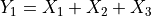and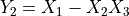for any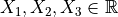.

The exact expectation and standard deviation of the output random variable are presented in the following table.

Variable

Expectation

Standard deviation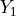0

1.732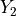0

1.415

import numpy as np
import openturns as ot

ot.Log.Show(ot.Log.NONE)


We first define the input random vector of the function.

X0 = ot.Normal(0.0, 1.0)
X1 = ot.Normal(0.0, 1.0)
X2 = ot.Normal(0.0, 1.0)
inputDistribution = ot.ComposedDistribution((X0, X1, X2))
inputRandomVector = ot.RandomVector(inputDistribution)


## The Python function¶

Based on a Python function defined with the def keyword, the PythonFunction class creates a function.

The simplest constructor of the PythonFunction class is:

PythonFunction ( nbInputs , nbOutputs , myPythonFunc )

where

• nbInputs: the number of inputs,

• nbOutputs: the number of outputs,

• myPythonFunc: a Python function.

The goal of the PythonFunction class are:

• to easily create a function in Python,

• use all the power of the Python libraries in order to evaluate the function.

The function mySimulator has the calling sequence y=mySimulator(x) where:

• x: the input of the function, a vector with nbInputs dimensions,

• y: the output of the function, a vector with nbOutputs dimensions.

def mySimulator(x):
y0 = x + x + x
y1 = x - x * x
y = [y0, y1]
return y


We now define the PythonFunction object.

myfunction = ot.PythonFunction(3, 2, mySimulator)


This function can be evaluated using parentheses. It produces the same outputs as the mySimulator function.

myfunction([1.0, 2.0, 3.0])


[6,-5]

However, the newly created myfunction has services that the basic Python function did not have. For example, we can create a CompositeRandomVector on top of it, by associating it to the input random vector.

outputVect = ot.CompositeRandomVector(myfunction, inputRandomVector)


In the following example, we estimate the output mean based on a simple Monte-Carlo experiment using 10000 function evaluations.

montecarlosize = 10000
outputSample = outputVect.getSample(montecarlosize)

empiricalMean = outputSample.computeMean()
print(empiricalMean)
empiricalSd = outputSample.computeStandardDeviation()
print(empiricalSd)

[-0.00555473,0.00220407]
[1.72426,1.40274]


## What types for x and for y ?¶

Not all types are possible for the inputs and outputs of the mySimulator function. The following table present some of the available types. All in all, any type which can be converted to or from a “vector” can be managed by the PythonFunction class.

Type

Input X

Output Y

list (Python)

NO

YES

tuple (Python)

NO

YES

array (NumPy)

NO

YES

Point (OpenTURNS)

YES

YES

## The vectorized Python function¶

The PythonFunction class has a func_sample option which vectorizes the computation so that all the output values in the sample are computed from a single function call, without any for loop. To make this possible, the input and output is then a Sample instead of a Point. This often boosts performance (but not always).

The calling sequence of a vectorized Python function is:

def mySimulator (x):

[…] return y

myfunction = PythonFunction(nbInputs, nbOutputs, func_sample = mySimulator)

where

• x: the input of the function, a Sample with size nbExperiments (getSize) and dimension nbInputs (getDimension),

• y: the output of the function

• a numpy array with nbExperiments rows and nbOutputs columns

• or a Sample with size nbExperiments and dimension nbOutputs

In the following, we present an vectorization example based on the numpy module.

def mySimulatorVect(x):
# Convert NumericalSample > Array Numpy
x = np.array(x)
x0 = x[:, 0]  # Extract column 0
x1 = x[:, 1]
x2 = x[:, 2]
y0 = x0 + x1 + x2
y1 = x0 - x1 * x2
# Stack the two rows
y = np.vstack((y0, y1))
y = y.transpose()
return y

myfunctionVect = ot.PythonFunction(3, 2, func_sample=mySimulatorVect)

outputVect = ot.CompositeRandomVector(myfunctionVect, inputRandomVector)

montecarlosize = 10000
outputSample = outputVect.getSample(montecarlosize)

empiricalMean = outputSample.computeMean()
print(empiricalMean)
empiricalSd = outputSample.computeStandardDeviation()
print(empiricalSd)

[-0.00134596,-0.00382747]
[1.75145,1.42678]


## How to manage the history¶

The MemoizeFunction class defines a history system to store the calls to the function.

Methods

Description

enableHistory()

enables the history (it is enabled by default)

disableHistory()

disables the history

clearHistory()

deletes the content of the history

getHistoryInput()

a Sample, the history of inputs X

getHistoryOutput()

a Sample, the history of outputs Y

myfunction = ot.PythonFunction(3, 2, mySimulator)
myfunction = ot.MemoizeFunction(myfunction)

outputVariableOfInterest = ot.CompositeRandomVector(myfunction, inputRandomVector)
montecarlosize = 10
outputSample = outputVariableOfInterest.getSample(montecarlosize)


Get the history of input points.

inputs = myfunction.getInputHistory()
inputs

v0 v1 v2 -0.2663513 -0.2534594 1.011153 1.283139 -0.5533954 -0.8628305 1.714011 0.8829328 0.05928211 0.02775571 1.239406 -3.246511 0.2525268 1.72599 -0.09525381 -0.3497864 -0.9771853 -0.6350077 -0.7103832 -0.9961178 0.8213179 -0.6293938 0.7427186 -0.1490467 -0.876391 -0.06694214 -0.2582989 -0.7666912 1.417515 0.564389

## Symbolic functions¶

The SymbolicFunction class can create symbolic functions whenever the function is simple enough to be expressed as a string.

This has at least two significant advantages.

• It generally improves the performance.

• This allows one to automatically evaluate the exact gradient and Hessian matrix.

In practice, some functions cannot be expressed as a symbolic function: in this case, the Python function cannot be avoided.

The calling sequence is the following:

 myfunction = SymbolicFunction( list_of_inputs, list_of_formulas)

where

• list_of_inputs: a list of nbInputs strings, the names of the input variables,

• list_of_formulas: a list of nbOutputs strings, the equations.

myfunction = ot.SymbolicFunction(("x0", "x1", "x2"), ("x0 + x1 + x2", "x0 - x1 * x2"))


A SymbolicFunction, like any other function, can also have a history.

myfunction = ot.MemoizeFunction(myfunction)

outputVect = ot.CompositeRandomVector(myfunction, inputRandomVector)

montecarlosize = 10000
outputSample = outputVect.getSample(montecarlosize)
empiricalMean = outputSample.computeMean()
print(empiricalMean)

[0.0341618,0.017369]


Since the history is enabled, we can get the history of outputs of the function.

outputs = myfunction.getOutputHistory()
outputs[1:10, :]

v0 v1 0.5508862 1.654515 -1.365358 -1.980954 -1.732063 -0.8032709 0.4673812 0.7841379 -2.50926 -1.470317 -0.1433779 -0.8622018 2.452678 -1.221384 -0.727263 4.544523 2.727623 3.208275